# Olympiad Test: Analytical Reasoning - 2

## 20 Questions MCQ Test Mathematical Olympiad Class 8 | Olympiad Test: Analytical Reasoning - 2

Description
Attempt Olympiad Test: Analytical Reasoning - 2 | 20 questions in 40 minutes | Mock test for Class 8 preparation | Free important questions MCQ to study Mathematical Olympiad Class 8 for Class 8 Exam | Download free PDF with solutions
QUESTION: 1

Solution:
QUESTION: 2

### Direction: In the following problems, count the number of squares in each figure.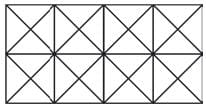Solution:

10 + 8 + 3 + 3 = 24

QUESTION: 3

### Direction: In the following problems, count the number of squares in each figure.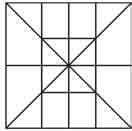Solution:

4 + 4 + 4 + 2 + 1 + 1 = 16

QUESTION: 4

Direction: In the following problems, count the number of squares in each figure.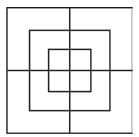Solution:
QUESTION: 5

Direction: In the following problems, count the number of squares in each figure.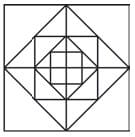Solution:
QUESTION: 6

Direction: In the following problems, count the number of squares in each figure.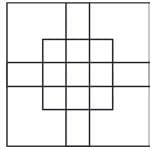Solution:
QUESTION: 7

Direction: In the following problems, find the number of parallelograms in each of the following figures.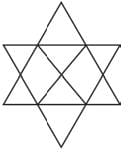Solution:

2 + 2 + 8 + 3 = 15.

QUESTION: 8

Direction: In the following problems, find the number of parallelograms in each of the following figures.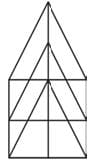Solution:
QUESTION: 9

Direction: In the following problems, find the number of parallelograms in each of the following figures.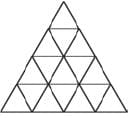Solution:
QUESTION: 10

Direction: In the following problems, find the number of parallelograms in each of the following figures.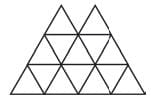Solution:
QUESTION: 11

Direction: In the following questions, what is the minimum number of straight lines ?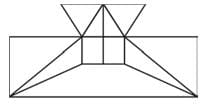Solution:
QUESTION: 12

Direction: In the following questions, what is the minimum number of straight lines ?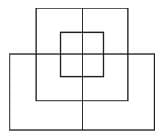Solution:
QUESTION: 13

Direction: In the following questions, what is the minimum number of straight lines ?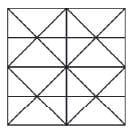Solution:
QUESTION: 14

Direction: In the following questions, what is the minimum number of straight lines ?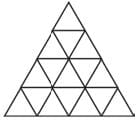Solution:
QUESTION: 15

Direction: In the following questions, what is the minimum number of straight lines ?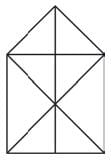Solution:
QUESTION: 16

Direction: In each of the following questions, what is the number of triangles and squares in the figure given below.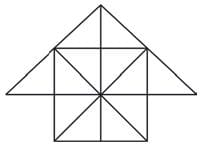Solution:
QUESTION: 17

Direction: In each of the following questions, what is the number of triangles and squares in the figure given below.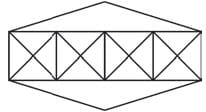Solution:
QUESTION: 18

Direction: In each of the following questions, what is the number of triangles and squares in the figure given below.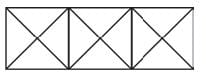Solution:
QUESTION: 19

Direction: In each of the following questions, what is the number of triangles and squares in the figure given below.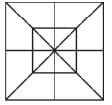Solution:
QUESTION: 20

Direction: In each of the following questions, what is the number of triangles and squares in the figure given below.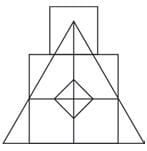Solution:Use Code STAYHOME200 and get INR 200 additional OFF Use Coupon Code